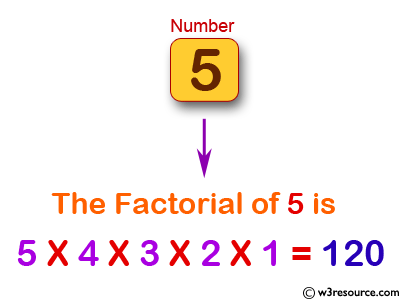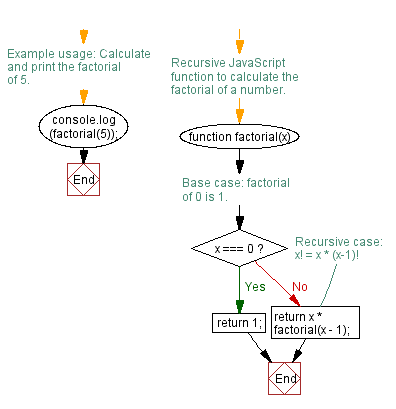# JavaScript: Calculate the factorial of a number

## JavaScript Function: Exercise-1 with Solution

Write a JavaScript program to calculate the factorial of a number.

In mathematics, the factorial of a non-negative integer n, denoted by n!, is the product of all positive integers less than or equal to n. For example, 5! = 5 x 4 x 3 x 2 x 1 = 120

Pictorial Presentation:Sample Solution:-

HTML Code:

``````<!DOCTYPE html>
<html>
<meta charset=utf-8 />
<title>Calculate the factorial of a number.</title>
<body>
</body>
</html>
```
```

JavaScript Code:

``````function factorial(x)
{

if (x === 0)
{
return 1;
}
return x * factorial(x-1);

}
console.log(factorial(5));
```
```

Output:

```120
```

Flowchart:Live Demo:

See the Pen javascript-recursion-function-exercise-1 by w3resource (@w3resource) on CodePen.

Improve this sample solution and post your code through Disqus

What is the difficulty level of this exercise?

Test your Programming skills with w3resource's quiz.

﻿

## JavaScript: Tips of the Day

Log the values that are commented out after the console.log statement

```function* startGame() {
const answer = yield 'Do you love JavaScript?';
if (answer !== 'Yes') {
return "Oh wow... Guess we're gone here";
}
return 'JavaScript loves you back ?';
}

const game = startGame();
console.log(/* 1 */); // Do you love JavaScript?
console.log(/* 2 */); // JavaScript loves you back ?
```

A generator function "pauses" its execution when it sees the yield keyword. First, we have to let the function yield the string "Do you love JavaScript?", which can be done by calling game.next().value.
Every line is executed, until it finds the first yield keyword. There is a yield keyword on the first line within the function: the execution stops with the first yield! This means that the variable answer is not defined yet!
When we call game.next("Yes").value, the previous yield is replaced with the value of the parameters passed to the next() function, "Yes" in this case. The value of the variable answer is now equal to "Yes". The condition of the if-statement returns false, and JavaScript loves you back ? gets logged.

Ref: https://bit.ly/3jFRBje